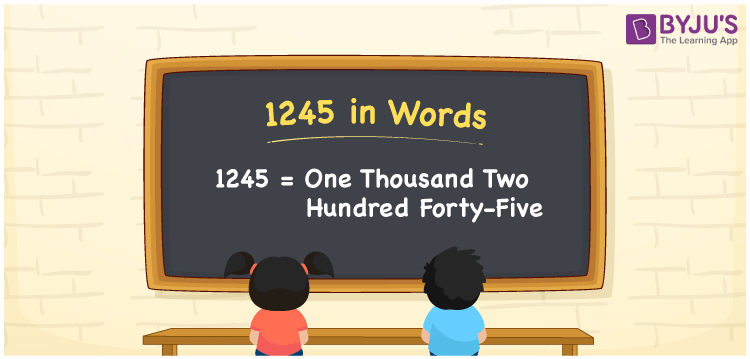# 1245 in Words

We can write 1245 in words as One thousand two hundred forty-five. If you spent Rs. 1245 to book a bus ticket, then you can say, “I spent One thousand two hundred forty-five rupees to book a bus ticket”. Thus, we can express the cardinal number 1245 in word form as “One thousand two hundred forty-five”.

 1245 in words One thousand two hundred forty-five One thousand two hundred forty-five in Numbers 1245

## 1245 in English Words

Using the English alphabet, we generally write numbers in words. Thus, we can spell 1245 in words as “One thousand two hundred forty-five”.## How to Write 1245 in Words?

The number 1245 can be converted into words using a place value chart with four columns, as shown below since it is a four-digit number.

 Thousands Hundreds Tens Ones 1 2 4 5

Here, ones = 5, tens = 4, hundreds = 2, and thousands = 1.

These numbers can be expanded as follows:

1 × Thousand + 2 × Hundred + 4 × Ten + 5 × One

= 1 × 1000 + 2 × 100 + 4 × 10 + 5 × 1

= 1000 + 200 + 40 + 5

= One thousand + Two hundred + Forty + five

= One thousand two hundred forty-five

Therefore, 1245 in words = One thousand two hundred forty-five.

As we know, 1245 is a natural number that precedes 1246 and succeeds 1244.

1245 in words – One thousand two hundred forty-five

Is 1245 an odd number? – Yes

Is 1245 an even number? – No

Is 1245 a prime number? – No

Is 1245 a composite number? – Yes

Is 1245 a perfect square number? – No

Is 1245 a perfect cube number? – No

## Frequently Asked Questions on 1245 in Words

Q1

### How do you write 1245 in words?

We can write the number 1245 in words as One thousand two hundred forty-five.
Q2

### How to write 1245 rupees in words on a cheque?

On a cheque, we generally write 1245 rupees in words as “One thousand two hundred forty-five rupees only”.
Q3

### What number is One thousand two hundred forty-five in words?

One thousand two hundred forty-five is equal to the number 1245. That means 1245 = One thousand two hundred forty-five